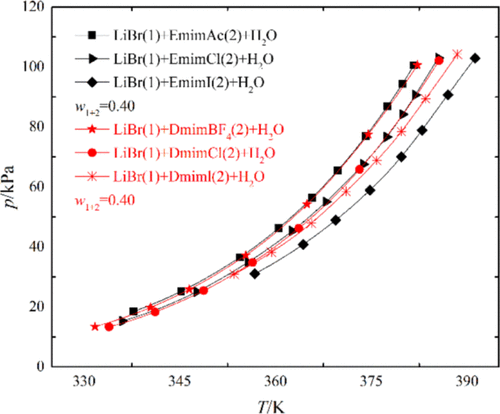Journal of Chemical & Engineering Data ( IF 2.298 ) Pub Date : 2020-01-07 , DOI: 10.1021/acs.jced.9b00614
Yongmei Xuan; Kaile Fang; Bosheng Duan; Neng Gao; Guangming ChenIonic liquids (ILs), 1-ethyl-3-methylimidazolium iodine [Emim]I and 1,3-dimethylimidazolium iodine [Dmim]I, were proposed as a novel potential solvent in traditional bromide aqueous solution (H2O + LiBr) in absorption refrigeration cycles. The vapor pressure data of two ternary systems H2O + LiBr + [Emim]I (mass ratio LiBr/[Emim]I = 4) at temperatures from 350.78 to 414.19 K and H2O + LiBr + [Dmim]I (mass ratio LiBr/[Dmim]I = 2) at temperatures from 350.69 to 409.63 K were reported by the boiling point method. The absorbent mass fractions are from 0.30 to 0.57 and 0.30 to 0.60, respectively. The measured data were correlated by using the Antoine-type equation, and the average absolute relative deviation (AARD %) between measured data and regression data was 0.54 and 0.39%. Compared with the vapor pressures of IL mixtures with the same organic cation, the vapor pressure order is LiBr + [Emim]I + H2O < LiBr + [Emim]Cl + H2O < LiBr + [Emim]Ac + H2O and LiBr + [Dmim]I + H2O < LiBr + [Dmim]Cl + H2O < LiBr + [Dmim]BF4 + H2O. Under experimental conditions, the hydrophilic properties of the iodine anion (I–) are better than those of the chlorine anion (Cl–), acetate anion (Ac–), and tetrafluoroborate anion (BF4–). Lower vapor pressure means stronger moisture absorption property. The proposed ternary mixtures have lower vapor pressures and can improve the absorption effect of the absorption system.

down
wechat
bug